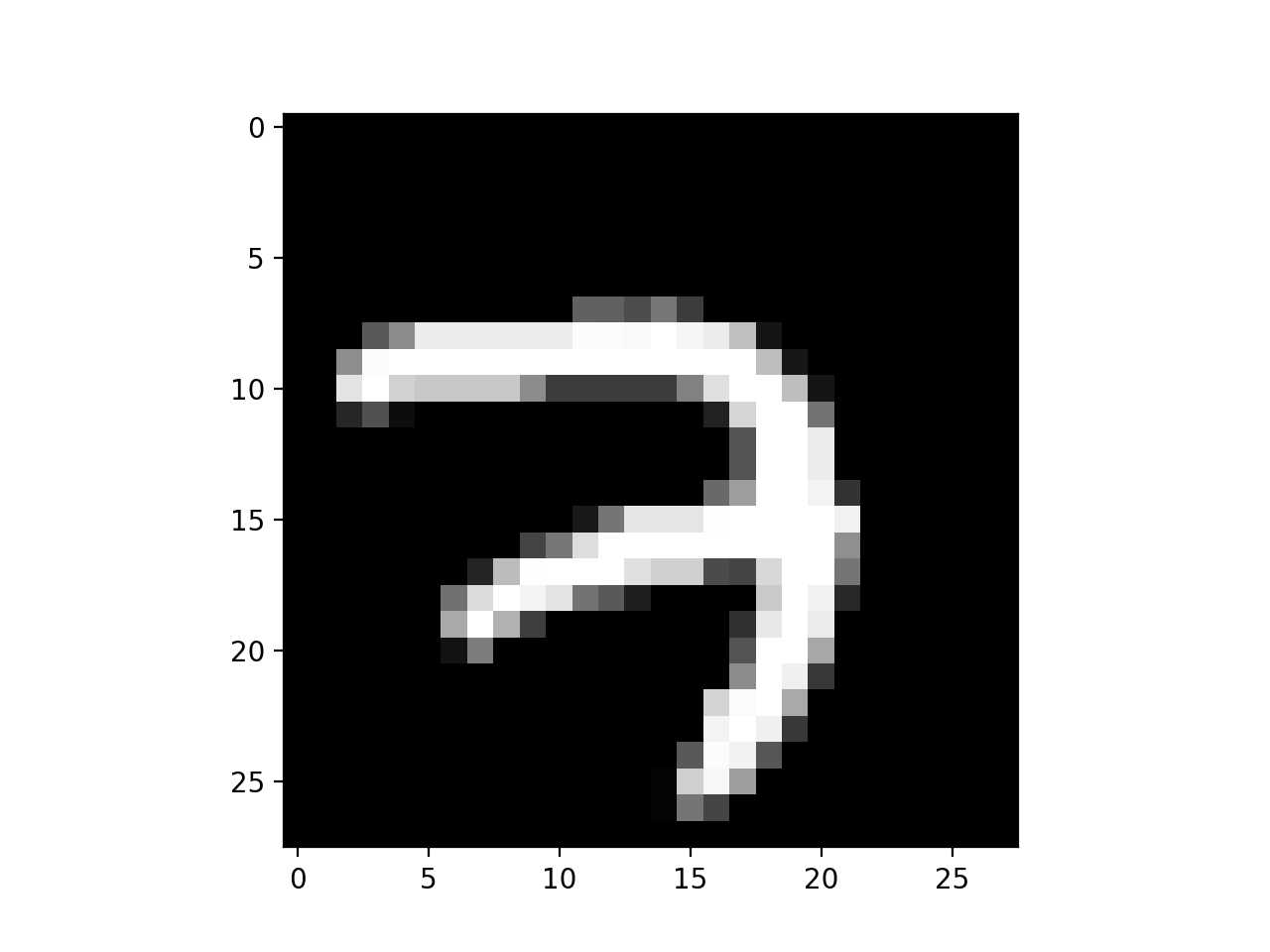# 卷积的发展历程，原理和基于 TensorFlow 的实现

#### 稀疏交互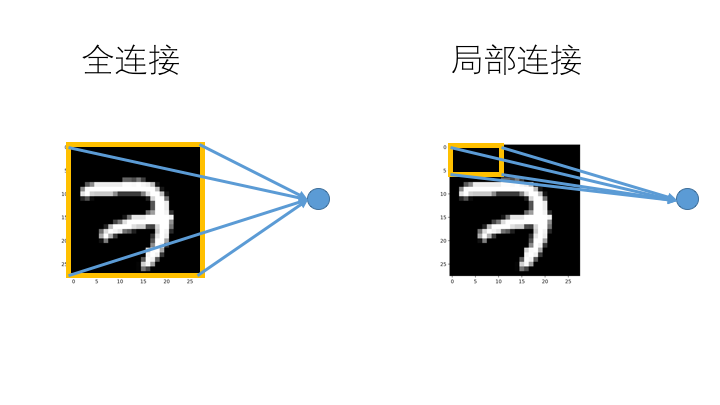##### 稀疏交互的实现

MNIST 原始图片：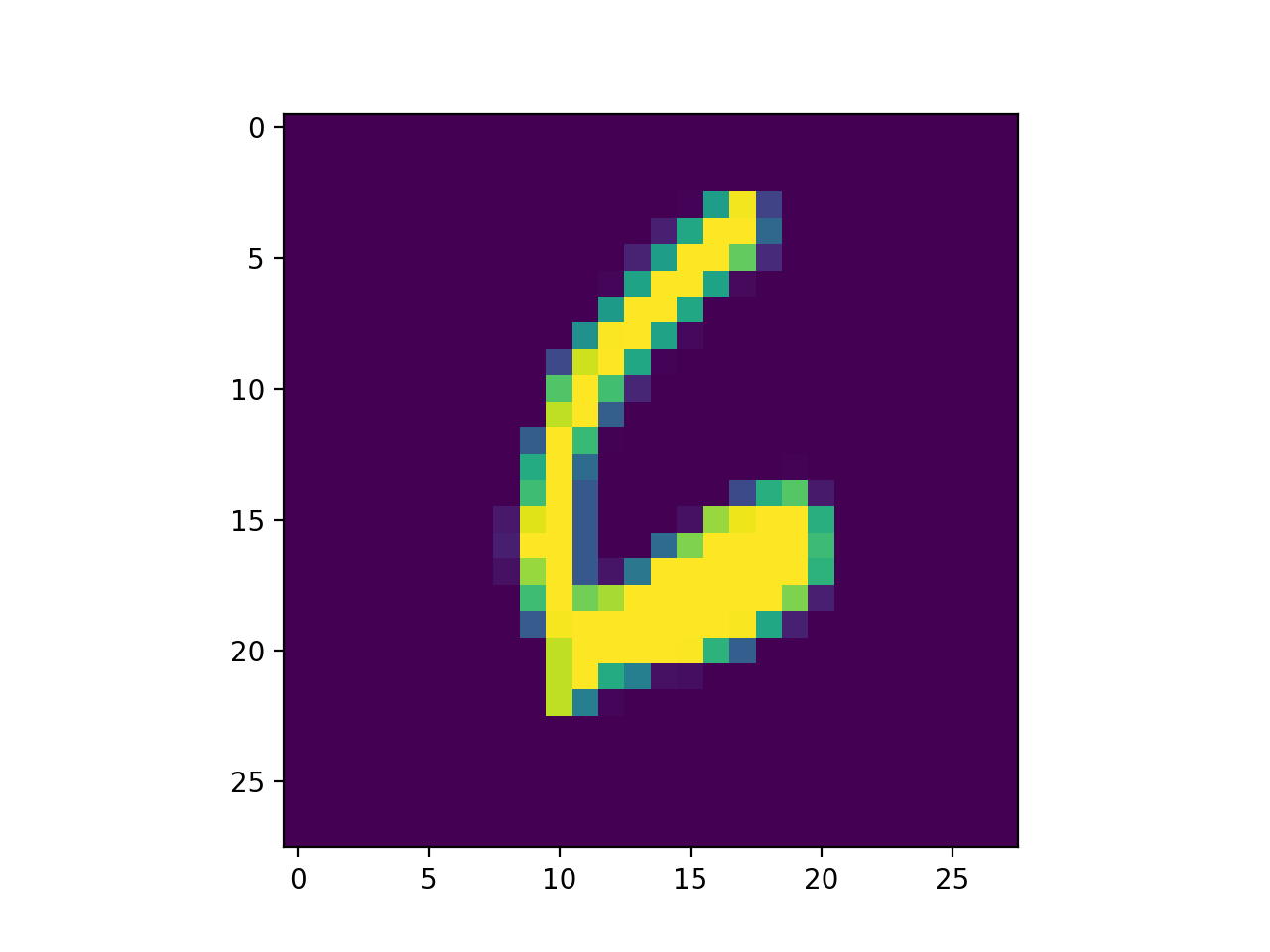1.局部区域的大小

2.局部特征的抽取次数

3.步长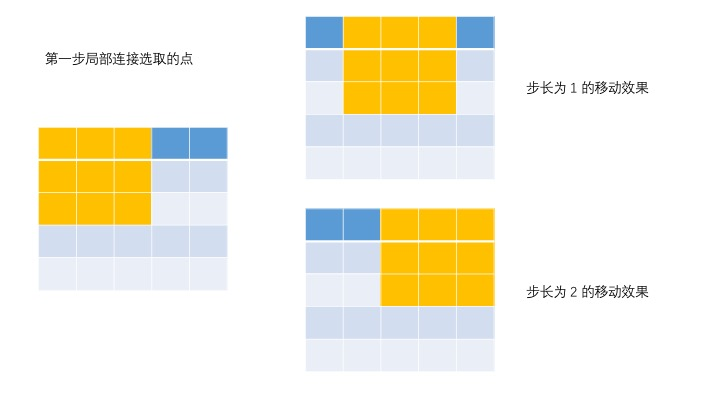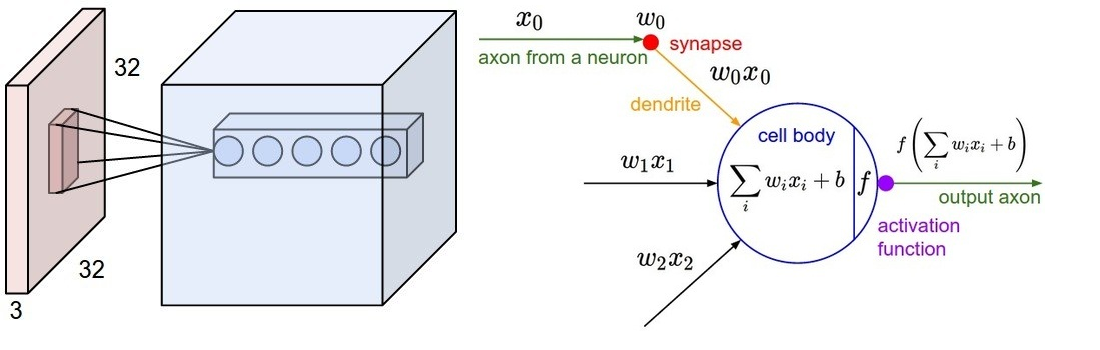4.边缘填充

4 * 4 的图像，进行了 3 * 3 的局部连接，维度发生了变化。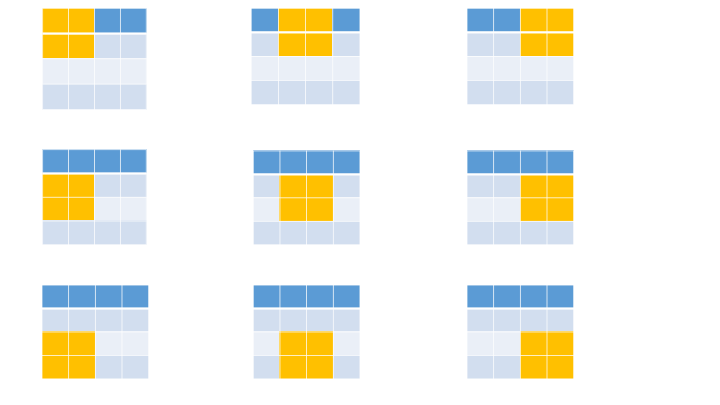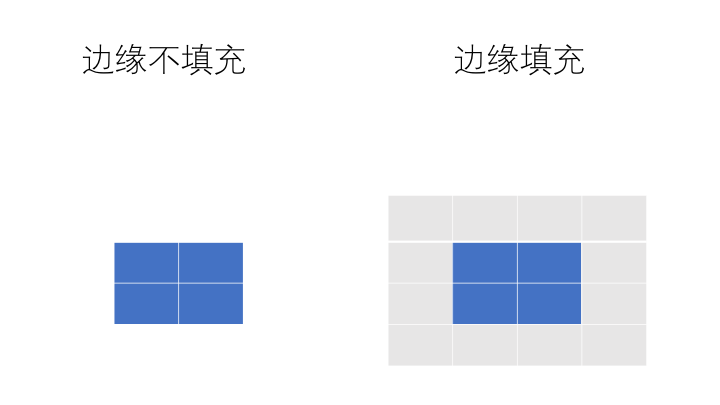#### TensorFlow 实现局部连接和权值共享

[code lang=text]
[[-1,-1,-1],
[-1,8,-1],
[-1,-1,-1]]
[/code]

[code lang=text]
import tensorflow as tf
import numpy as np
from tensorflow.examples.tutorials.mnist import input_data
import matplotlib.pyplot as plt

#引入 MNIST 数据集

#选取训练集中的第 1 个图像的矩阵
mnist_one=mnist.train.images

plt.subplot(121)
plt.imshow(mnist_one.reshape((28,28)), cmap=plt.cm.gray)

#输出图片的维度，结果是：(784,)
print(mnist_one.shape)

#因为原始的数据是长度是 784 向量，需要转换成 1*28*28*1 的矩阵。
mnist_one_image=mnist_one.reshape((1,28,28,1))

#输出矩阵的维度
print(mnist_one_image.shape)

#滤波器参数
filter_array=np.asarray([[-1,-1,-1],[-1,8,-1],[-1,-1,-1]])

#滤波器维度
print(filter_array.shape)

#调整滤波器维度
filter_tensor=filter_array.reshape((3,3,1,1))

#卷机操作

#返回的张量维度
print(conv_image_tensor.shape)

#调整为二维图片
conv_image=tf.reshape(conv_image_tensor,[28,28])

with tf.Session() as sess:

#获得张量的值
conv_image=sess.run(conv_image)

plt.subplot(122)

#使用 matplotlib 输出为图片
plt.imshow(conv_image, cmap=plt.cm.gray)

plt.show()
[/code]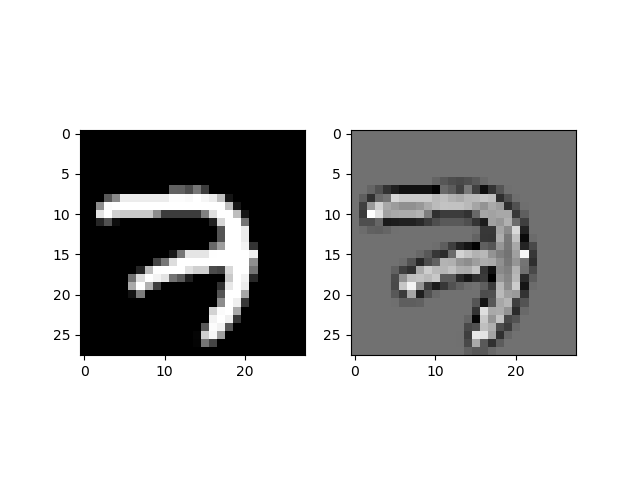### 池化介绍

#### 池化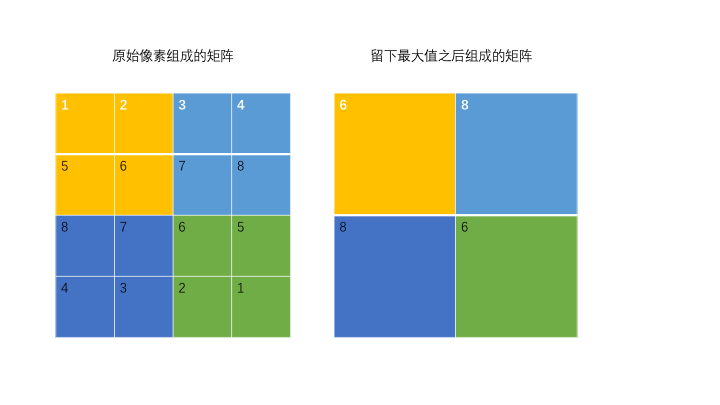### 手写数字识别

#### 数据准备

• MNIST 数据集下载

MNIST 数据集可以从 THE MNIST DATABASE of handwritten digits 的网站直接下载。

train-images-idx3-ubyte.gz: 训练集图片
train-labels-idx1-ubyte.gz: 训练集列标
t10k-images-idx3-ubyte.gz: 测试集图片
t10k-labels-idx1-ubyte.gz: 测试集列标

TensorFlow 有加载 MNIST 数据库相关的模块，可以在程序运行时直接加载。

[code lang=text]
from tensorflow.examples.tutorials.mnist import input_data
import matplotlib.pyplot as pyplot

#引入 MNIST 数据集

#选取训练集中的第 1 个图像的矩阵
mnist_one=mnist.train.images

#输出图片的维度，结果是：(784,)
print(mnist_one.shape)

#因为原始的数据是长度是 784 向量，需要转换成 28*28 的矩阵。
mnist_one_image=mnist_one.reshape((28,28))

#输出矩阵的维度
print(mnist_one_image.shape)

#使用 matplotlib 输出为图片
pyplot.imshow(mnist_one_image)

pyplot.show()
[/code]

1.单个手写数字图片的维度：
(784,)

2.转化为二维矩阵之后的打印结果：
(28, 28)

3.使用 matplotlib 输出为图片